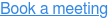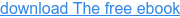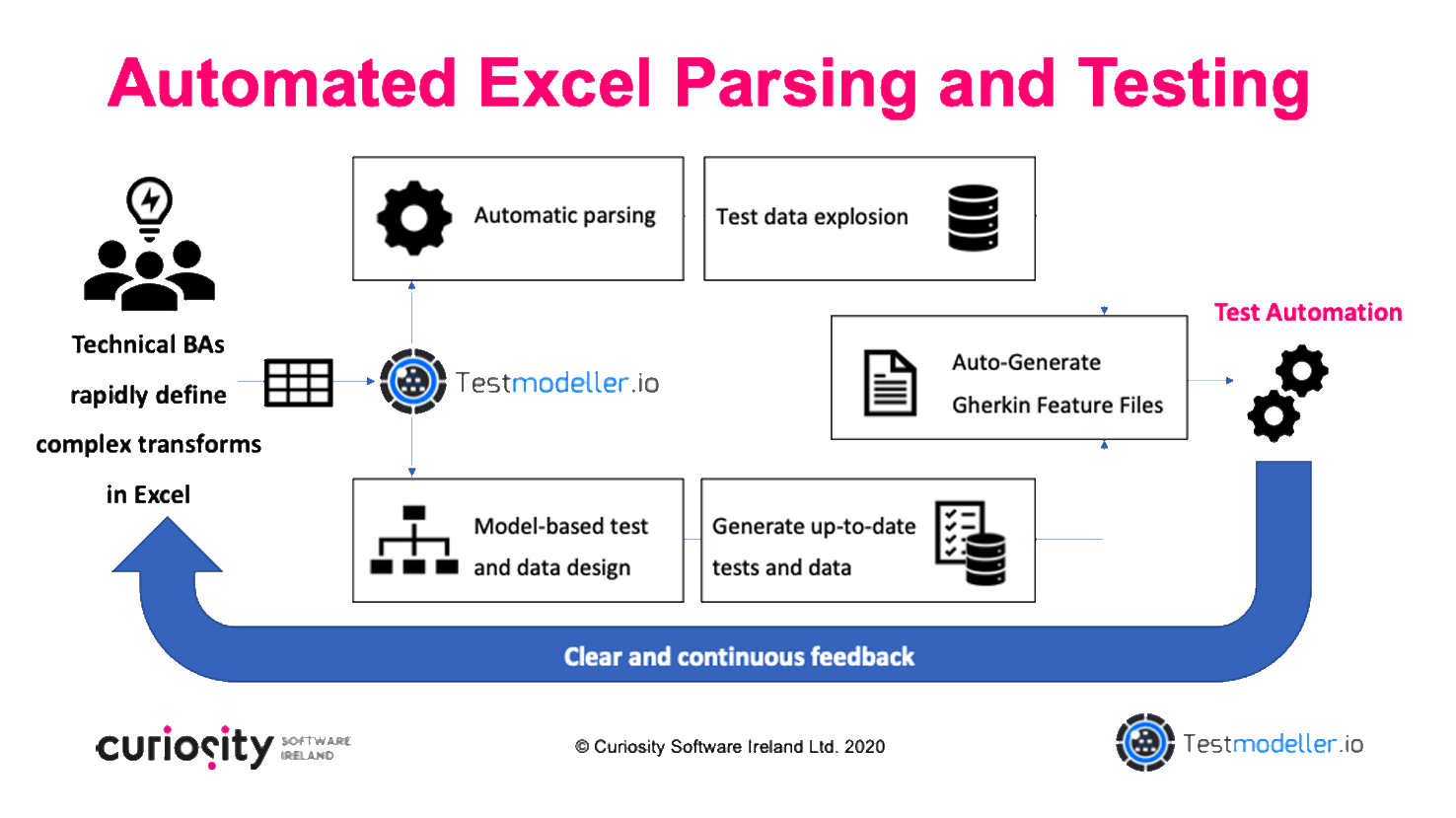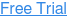# Excel Formula Parsing

Rapidly understand, document and test complex data transforms

Replace complex Excel spreadsheets with visual flowcharts and easy-to-read formula, complete with rich test data and automated tests. Test Modeller automatically parses formulas defined in Excel, allowing BAs and QA teams to collaborate as they design and rigorously test transforms in-sprint.## A ‘simple’ solution produces massive testing complexity

Excel remains a tool of choice when designing transforms for business-critical data. Technical BAs today frequently construct complex transforms in intricate spreadsheets, combining Excel and SQL functions. These complex transforms require rigorous testing, but manually deriving test scenarios from the dense spreadsheets is slow and error prone. It misses many logical scenarios buried in the sprawling sheets, creating low coverage tests that leave transforms exposed to bugs. QA further requires a rich spread of test data values, each with accurately defined expected results. Often, neither is available, and QA teams instead feed in a small range of values, guessing at expected results.## Take the complexity out of testing complex formulas

Test Modeller provides a rapid and reliable approach to understanding complex Excel formula, while automatically generating test scenarios with matching automated tests. The model-based approach further documents complex logic in easy-to-understand visual flowcharts, allowing BAs and QA teams to collaborate closely when design and rigorously testing business-critical transforms.Test Modeller automatically parses complex Excel spreadsheets, breaking formula down into logical steps. An easy-to-use spreadsheet then allows QA teams to pass data through each step, verifying that the formulas produce the right result at each point. Two approaches in turn test the complex formula rigorously. First, a Brute Force Methodology which explodes the coverage of specified data values, recombining them to feed a rich spread of values through the formula. Alternatively, a model-based based approach models the formulas as logical flowcharts, applying automated coverage algorithms to generate a complete spread of data. Executing the rich data is quick and easy with Test Modeller, which automatically converts the scenarios into Gherkin Feature Files to drive rigorous test automation.## Rapidly understand, document and test complex data transforms

Watch this short example of Excel parsing and testing, to discover how:

1. Test Modeller automatically parses formulas stored across Excel spreadsheets, clearly breaking formula down into their logical steps and proposing seed test data.

2. Manually entering seed data into the spreadsheet provides a quick method to verify the parsing, while validating that the formulas produce the right result at each logical step.

3. Automated pairwise techniques automatically combine the seed data for a rapid “Brute Force Methodology”, quickly producing a spread of data to test the Excel formulas.

4. Test Modeller automatically creates visual PNG diagrams of the tested formula, displaying the logic of the formula far more clearly than in intricate Excel spreadsheets.

5. The automated Excel parsing groups logically distinct scenarios, allowing QA teams to rapidly select the smallest number of scenarios needed for comprehensive testing.

6. Visual flowchart modelling breaks formula down into their core logic, overlaying Excel-like functions and dynamically allocating data from back-end databases for rigorous testing.

7. A range of automated coverage algorithms automatically generate the optimal number of paths through the modelled logic, generating a comprehensive test suite with complete test data.

8. Test Modeller automatically converts the complete test scenarios and data into Gherkin Feature Files, ready to export to IDEs and test management tools for rapid automated testing.